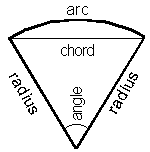## Radius Angle Arc Chord Area of A Sector Calculator

 radius units angle degrees arc units chord units area square units

Significant figures:

A sector ia a part of a circle. It has two lines and a arc forms the circumference of it. If the angle of the sector is 180 degrees the sector is a semi-circle, the angle of the sector is 360 degrees then the sector is a circle.